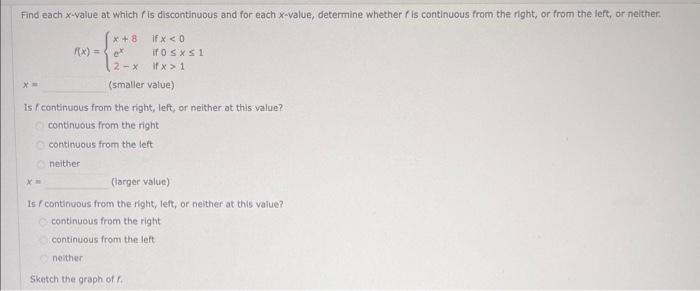Home / Expert Answers / Calculus / find-each-x-value-at-which-f-is-discontinuous-and-for-each-x-value-determine-whe-pa731

# (Solved): Find each $$x$$-value at which $$f$$ is discontinuous and for each $$x$$-value, determine whe ...Find each $$x$$-value at which $$f$$ is discontinuous and for each $$x$$-value, determine whether $$f$$ is continuous from the right, or from the left, or nelther. $f(x)=\left\{\begin{array}{ll} x+8 & \text { if } x<0 \\ e^{x} & \text { if } 0 \leq x \leq 1 \\ 2-x & \text { if } x>1 \end{array}\right.$ (smaller value) Is f continuous from the right, left, or neither at this -value? continuous from the right continuous from the left neither $$x=\quad$$ (targer value) Is f continuous from the fight, feft, or neither at this value? continuous from the right. continuous from the left neither Sketch the graph of $$f$$.

We have an Answer from Expert